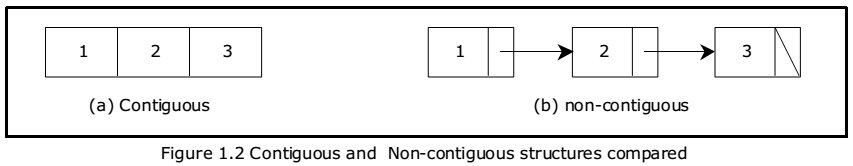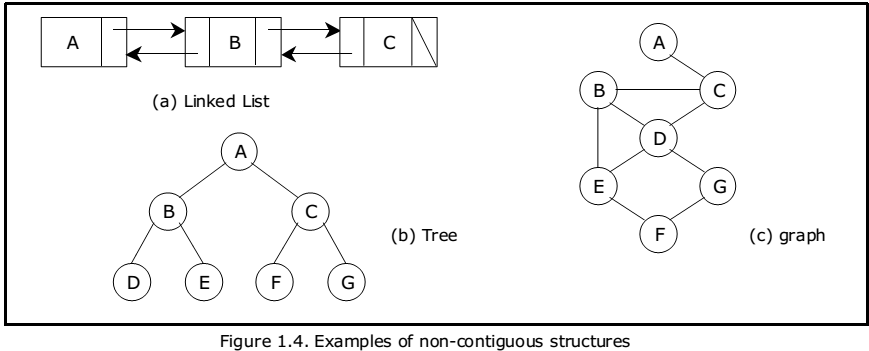What is mean by data structure ?
Lets have brief introduction about data structure, Lets us takes an example ,in our room we have different things but scattered in different way, if we can place those things in proper way ,we can find the things in fastest way and in proper time…The Same way Data structure is the way we need to organize the data, so that it can be used effectively by the program. So you are all familiar with certain data structures, an array or a list for instance.

Manipulation involves ,Adding, searching,Deleting and Rearranging data items

Data structures are divided into two types:

•  Primitive data structures.
•  Non-primitive data structures.

Primitive Data Structures are the basic data structures that directly operate upon the machine instructions. They have different representations on different computers.

1. Int:Integers
2. Float: floating point numbers
3. Char: Character constants
4. Str: string constants
5. Pointer

Non-primitive data structures are more complicated data structures and are derived from primitive data structures. They emphasize on grouping same or different data items with relationship between each data item.
Type of Non-Primitive Data Structures

• Arrays
• lists
1.Linear List:a data structure which organizes data elements in a sequence that is one after the other is referred to as linear List,array is a common example of linear data structure that exhibit linearity
For Example:Stacks and Queues
2.Non-Linear Lists
For Example :Graphs and Trees
• files

Lets Also Understand The Term Algorithm, What is algorithm?

The information contained in a data structure is manipulated by a systematic and step-by-step process which is called algorithm

Data structures: Organization of data

The collection of data you work with in a program have some kind of structure or organization. No matter how complex your data structures are they can be broken down into two fundamental types:

•  Contiguous
•  Non-Contiguous

Contiguous

In contiguous structures, terms of data are kept together in memory (either RAM or in a file). An array is an example of a contiguous structure. Since each element in the array is located next to one or two other elements.

non-contiguous structure

In a non-contiguous structure Items were scattered in memory, but we linked to each other in some way. A linked list is an example of a non-contiguous data structure. Here, the nodes of the list are linked together using pointers stored in each node. For Better Understanding check the figure below .Contiguous structures:

Contiguous structures can be broken drawn further into two kinds:
those that contain data items of all the same size, and those where the size may differ. The first kind is called the array. Figure 1.3(a) shows an example of an array of numbers. In an array, each element is of the same type, and thus has the same size.

The second kind of contiguous structure is called structure, figure 1.3(b) shows a simple structure consisting of a person’s name and age. In a struct, elements may be of different data types and thus may have different sizes.

For example, a person’s age can be represented with a simple integer that occupies two bytes of memory. But his or her name, represented as a string of characters, may require many bytes and may even be of varying length.Non-contiguous structures:

Non-contiguous structures are implemented as a collection of data-items, called nodes, where each node can point to one or more other nodes in the collection. The simplest kind of noncontiguous structure is linked list.

A linked list represents a linear, one-dimension type of non-contiguous structure, where there is only the notation of backwards and forwards. A tree such as shown in figure 1.4(b) is an example of a two-dimensional non-contiguous structure. Here, there is the notion of up and down and left and right

In a tree each node has only one link that leads into the node and links can only go down the tree. The most general type of non-contiguous structure, called a graph has no such restrictions. Figure 1.4(c) is an example of a graph.Hybrid structures:

If two basic types of structures are mixed then it is a hybrid form. Then one part contiguous and another part non-contiguous.

Abstract Data Type (ADT):

For example, a stack is a typical abstract data type. Items stored in a stack can only be added and removed in certain order – the last item added is the first item removed. We call these operations, pushing and popping. In this definition, we haven’t specified have items are stored on the stack, or how the items are pushed and popped.

For example, if we want to read a file, we wrote the code to read the physical file device. That is, we may have to write the same code over and over again. So we created what is known today as an ADT. We wrote the code to read a file and placed it in a library for a programmer to use.

As another example, the code to read from a keyboard is an ADT. It has a data structure, character and set of operations that can be used to read that data structure.

Selecting a data structure to match the operation:
The most important process in designing a problem involves choosing which data structure to
use. The choice depends greatly on the type of operations you wish to perform.

Suppose we have an application that uses a sequence of objects, where one of the main
operations is delete an object from the middle of the sequence. The code for this is as follows:

```void delete (int *seg, int &n, int posn)
// delete the item at position from an array of n elements.
{
if (n)
{
int i=posn;
n--;
while (i < n)
{
seq[i] = seg[i+1];
i++;
}
}
return;
}```

This function shifts towards the front all elements that follow the element at position posn. This shifting involves data movement that, for integer elements, which is too costly. However, suppose the array stores larger objects, and lots of them. In this case, the overhead for moving data becomes high. The problem is that, in a contiguous structure, such as an array the logical ordering (the ordering that we wish to interpret our elements to have) is the same as the physical ordering (the ordering that the elements actually have in memory).

If we choose non-contiguous representation, however we can separate the logical ordering from the physical ordering and thus change one without affecting the other. For example, if we store our collection of elements using a double–linked list (with previous and next pointers), we can do the deletion without moving the elements, instead, we just modify the pointers in each node. The code using double linked list is as follows:

```void delete (node * beg, int posn)
//delete the item at posn from a list of elements.
{
int i = posn;
node *q = beg;
while (i && q)
{i--;
q = q Æ next;
}
if (q)
{ /* not at end of list, so detach P by making previous and
next nodes point to each other */
node *p = q -> prev;
node *n = q -> next;
if (p)
p -> next = n;
if (n)
n -> prev = P;
}
return;
}```

The process of detecting a node from a list is independent of the type of data stored in the
node, and can be accomplished with some pointer manipulation

Some Important Things to be noted about lined lists and Array:
Since very little data is moved during this process, the deletion using linked lists will often be faster than when arrays are used.

It may seem that linked lists are superior to arrays. But is that always true? There are trade offs. Our linked lists yield faster deletions, but they take up more space because they require two extra pointers per element.

You may be interested in:
Data Structures and Algorithms – MCQs.
Data Structures and Algorithms Online Tests